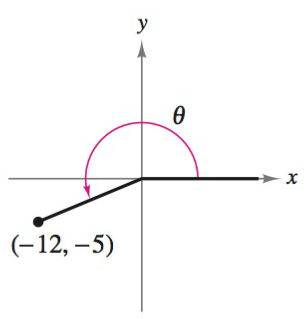Chapter 8.2, Problem 3E### Calculus: An Applied Approach (Min...

10th Edition
Ron Larson
ISBN: 9781305860919

#### Solutions

Chapter
Section### Calculus: An Applied Approach (Min...

10th Edition
Ron Larson
ISBN: 9781305860919
Textbook Problem
1 views

# Evaluating Trigonometric Functions In Exercises 1-6, find the exact values of the six trigonometric functions of the angle θ. See Example 1.To determine

To calculate: The exact values of the six trigonometric functions of the angle θ for the provided figure.Explanation

Given Information:

The figure provided below shows the angle θ.

Formula used:

The trigonometric functions are,

sinθ=yr         cscθ=rycosθ=xr         secθ=rxtanθ=yx         cotθ=xy

Where, x is the length of the side adjacent to θ, y is the length of the side opposite to θ, and r=x2+y2 is the length of hypotenuse r0.

Calculation:

From the provided figure, the value of x is 12 and y is 5.

Substitute x=12 and y=5 in the equation r=x2+y2.

r=(12)2+(5)2=144+25=169=13

Substitute y=5 and r=13 in sinθ=yr.

sinθ=513

Substitute x=12 and r=13 in cosθ=xr

### Still sussing out bartleby?

Check out a sample textbook solution.

See a sample solution

#### The Solution to Your Study Problems

Bartleby provides explanations to thousands of textbook problems written by our experts, many with advanced degrees!

Get Started

#### In Exercises 2336, find the domain of the function. 29. f(x)=5x

Applied Calculus for the Managerial, Life, and Social Sciences: A Brief Approach

#### j × (−k) = i −i j + k −j − k

Study Guide for Stewart's Multivariable Calculus, 8th

#### f(x) = (x2 3)2 has points of inflection at x = _____. a) 0,3,3 b) 1, 1 c) 0, 1, 1 d) 3,3

Study Guide for Stewart's Single Variable Calculus: Early Transcendentals, 8th